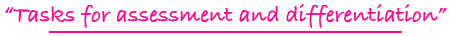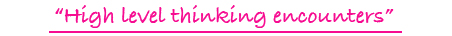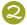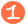•••Tiling Task Card Packets include 20 unique task cards on card stock,  3 backdrop cards , tracking sheets, suggestions and answer sheets.  These are contained in a printed manila envelope for convenient storing.  A set of number tiles 0 - 9 is  needed for each task card

2 - 4

Challenge Level:PDF FORMAT
(You will receive both color and black and white files for printing.)\$10.00

4 - 8

2 X 1 MULTIPLICATION TILES

multiplying 2-digit numbers by a single-digit number to yield a 2-digit or 3-digit product

Challenge Level:\$15.00

4 - 8

2 X 1 MULTIPLICATION TILES DIGITAL

multiplying 2-digit numbers by a single-digit number to yield a 2-digit or 3-digit product

Challenge Level:DIGITAL FORMAT\$10.00

4 - 8

2 X 1 MULTIPLICATION TILES

multiplying 2-digit numbers by a single-digit number to yield a 2-digit or 3-digit product

Challenge Level:PDF FORMAT
(You will receive both color and black and white files for printing.)\$10.00

2 - 5

2-DIGIT TILES: PLACE VALUE AND +/-

forming 2-digit numbers which meet certain constraints and dealing with the plus/minus symbol for addition and subtraction of 2-digit numbers

Challenge Level:\$15.00

5 - 8

UNKNOWN TILES: X Y Z

finding the value of 3 variables by employing logical and algebraic thinking to addition problems

Challenge Level:\$15.00

3 - 6

UP AND DOWN TILES DIGITAL

adding and subtracting positive and negative numbers by visualizing a thermometer with numbers above zero and below zero

Challenge Level:DIGITAL FORMAT\$10.00

3 - 6

UP AND DOWN TILES

adding and subtracting positive and negative numbers by visualizing a thermometer with numbers above zero and below zero

Challenge Level:PDF FORMAT
(You will receive both color and black and white files for printing.)\$10.00

2 - 5

VALUE TILES

forming values including money, place value, equalities, and inequalities

Challenge Level:\$15.00

2 - 5

VALUE TILES DIGITAL

forming values including money, place value, equalities, and inequalities

Challenge Level:DIGITAL FORMAT\$10.00

2 - 5

VALUE TILES

forming values including money, place value, equalities, and inequalities

Challenge Level:PDF FORMAT
(You will receive both color and black and white files for printing.)\$10.00

4 - 8

VARIABLE TILES DIGITAL

finding possible values for x and y or a and b when given an equation with 2 unknowns; if...then... statement and T table organization are utilized

Challenge Level:DIGITAL FORMAT\$10.00

4 - 8

VARIABLE TILES

finding possible values for x and y or a and b when given an equation with 2 unknowns; if...then... statement and T table organization are utilized

Challenge Level:PDF FORMAT
(You will receive both color and black and white files for printing.)\$10.00

3 - 6

VIEW A FRACTION TILES DIGITAL

forming common fractions from pictures, including equivalent fractions and comparisons of fractions

Challenge Level:DIGITAL FORMAT\$10.00

3 - 6

VIEW A FRACTION TILES

forming common fractions from pictures, including equivalent fractions and comparisons of fractions

Challenge Level:PDF FORMAT
(You will receive both color and black and white files for printing.)\$10.00

1 - 3

seeing addition and subtraction representations with black and white circles and writing horizontal addition and subtraction equations

Challenge Level:\$15.00

1 - 3

VIEWING ADDITION & SUBTRACTION TILES DIGITAL

seeing addition and subtraction representations with black and white circles and writing horizontal addition and subtraction equations

Challenge Level:DIGITAL FORMAT\$10.00

1 - 3

seeing addition and subtraction representations with black and white circles and writing horizontal addition and subtraction equations

Challenge Level:PDF FORMAT
(You will receive both color and black and white files for printing.)\$10.00

5 - 8

VISUALIZING TILES

dealing with representational models for common fractions, percent, ratio, and square numbers

Challenge Level:\$15.00

5 - 8

WATCH THE ORDER EQUATION TILES

forming balanced equations plus following the rules for the order of operations; equations may balance to a positive or negative whole number or to a common fraction

Challenge Level:\$15.00